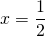# Solution assignment 06 The basics

### Assignment 6

Solve the following equation: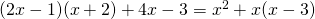### Solution

At first sight this seems a quadratic equation because both the left- and right-hand side contain a square. However, this is not the case. If we work out the formula the squares disappear: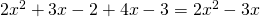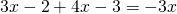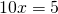and thus: